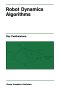# Physics - Inertia

Here:
https://www.euclideanspace.com/physics/dynamics/inertia/index.htm

You are discussing the inertia tensor. You have these lines about halfway down
the page:

T = F x s (force times distance, x is the cross product)

substuting F=m a gives,

T = m * s x a

therefore defining h = m * s gives

T = h x a

Here:

T = F x s

is incorrect. The correct equation is T = R X F where R is the distance vector.
This would make the rest of your derivation correct. Starting with T = F X
s and then ending with T = m*s X a would imply that the cross product is communitive,
which it is not.

I have enjoyed the site so far, Thanks

Israel

 metadata block see also: Correspondence about this page Book Shop - Further reading. Where I can, I have put links to Amazon for books that are relevant to the subject, click on the appropriate country flag to get more details of the book or to buy it from them.Game Physics - This book has some useful stuff, its more of a textbook, not a step by step guide (although it does have a disc with a lot of C++ code). About the first third of the book is a physics textbook with theoretical exercises, the middle bit covers physics engine topics, and the last third of the book covers mathematical topics. I think I would use this book as a reference book to lookup the theory behind something I might be working on rather than a book to work through in order.Robot Dynamics Algorithms (Kluwer International Series in Engineering and Computer Science, 22) Commercial Software Shop Where I can, I have put links to Amazon for commercial software, not directly related to the software project, but related to the subject being discussed, click on the appropriate country flag to get more details of the software or to buy it from them.Mathmatica

This site may have errors. Don't use for critical systems.